## Cross Reference Down

#### Nagasaka, K (1984). On Benford's Law. Annals of the Institute of Statistical Mathematics 36(2), pp. 337-352.

This work cites the following items of the Benford Online Bibliography:

 Benford, F (1938). The law of anomalous numbers. Proceedings of the American Philosophical Society, Vol. 78, No. 4 (Mar. 31, 1938), pp. 551-572.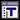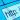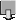Brady, WG (1978). More on Benford’s law. Fibonacci Quarterly 16(1), pp. 51-52.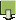Brown, JR and Duncan, RL (1970). Modulo one uniform distribution of the sequence of logarithms of certain recursive sequences. Fibonacci Quarterly 8, pp. 482-486. ISSN/ISBN:0015-0517.Diaconis, P (1977). The Distribution of Leading Digits and Uniform Distribution Mod 1. Annals of Probability 5(1), pp. 72-81. ISSN/ISBN:0091-1798.Duncan, RL (1967). An application of uniform distributions to the Fibonacci numbers. Fibonacci Quarterly 5, pp. 137-140.Flehinger, BJ (1966). On the Probability that a Random Integer has Initial Digit A. American Mathematical Monthly 73(10), pp. 1056-1061. ISSN/ISBN:0002-9890. DOI:10.2307/2314636.Furry, WH and Hurwitz, H (1945). Distribution of numbers and distribution of significant figures. Nature 155(3924), pp. 52-53. DOI:doi:10.1038/155052a0.Goudsmit, SA and Furry, WH (1944). Significant figures of numbers in statistical tables. Nature 154(3921), pp. 800-801. ISSN/ISBN:0028-0836. DOI:10.1038/154800a0.Kuipers, L (1969). Remark on a paper by R.L. Duncan concerning the uniform distribution mod 1 of the sequence of the logarithms of the Fibonacci numbers. Fibonacci Quarterly 7, pp. 465-466, 473.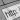Kuipers, L and Niederreiter, H (1974). Uniform Distribution of Sequences. J. Wiley; newer edition - 2006 from Dover. ISSN/ISBN:0486450198.Kuipers, L and Shiue, JS (1973). Remark on a paper by Duncan and Brown on the sequence of logarithms of certain recursive sequences. Fibonacci Quarterly 11(3), pp. 292-294.Pinkham, RS (1961). On the Distribution of First Significant Digits. Annals of Mathematical Statistics 32(4), pp. 1223-1230. ISSN/ISBN:0003-4851.Raimi, RA (1969). On Distribution of First Significant Figures. American Mathematical Monthly 76(4), pp. 342-348. ISSN/ISBN:0002-9890. DOI:10.2307/2316424.Raimi, RA (1976). The First Digit Problem. American Mathematical Monthly 83(7), pp. 521-538. ISSN/ISBN:0002-9890. DOI:10.2307/2319349.Washington, LC (1981). Benford’s law for Fibonacci and Lucas numbers. Fibonacci Quarterly 19, 175-177.Wlodarski, J (1971). Fibonacci and Lucas Numbers tend to obey Benford’s law. Fibonacci Quarterly 9, 87-88.